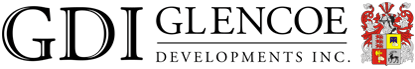Townhouse + Condo Living## Current Developments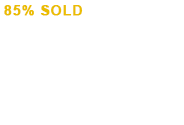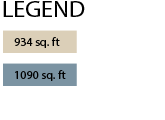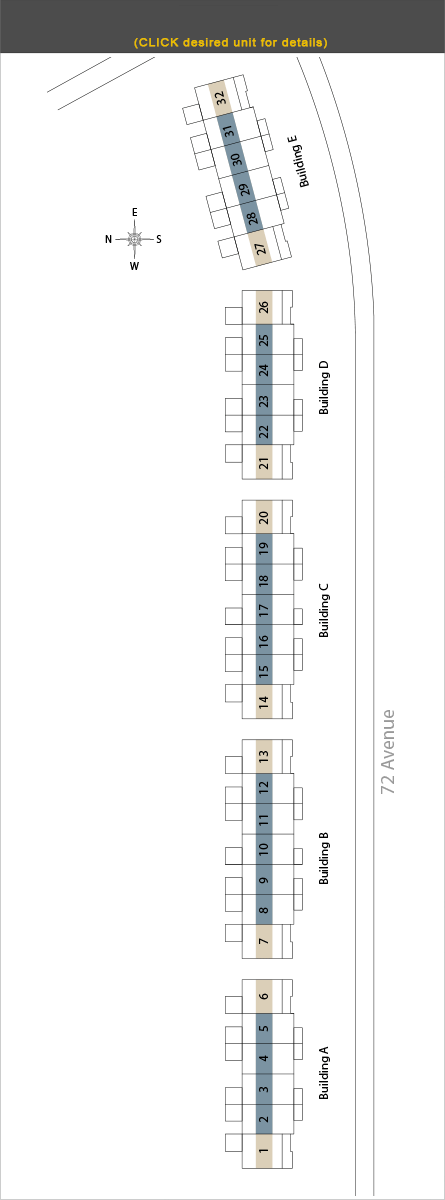[x] close[x] close[x] close[x] close[x] close[x] close[x] close[x] close[x] close[x] close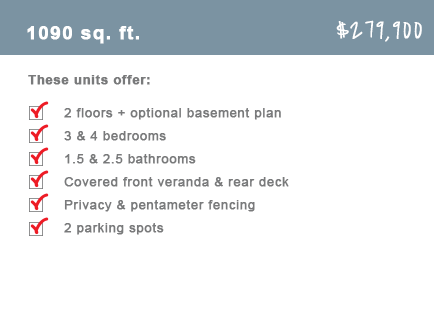[x] close[x] close[x] close[x] close[x] close[x] close[x] close[x] close[x] close[x] close[x] close[x] close[x] close[x] close[x] close[x] close[x] close[x] close[x] close[x] close[x] close[x] close